# Soret and Dufour Effects on the Boundary Layer Magneto-Fluid Flow Past an Infinite Vertical Porous Plate

Soret and Dufour Effects on the Boundary Layer Magneto-Fluid Flow Past an Infinite Vertical Porous Plate
Department of Mathematics, Faculty of Civil Engineering, Khulna University of Engineering & Technology, Khulna 9203, Bangladesh

Corresponding Author Email:
mmhasanbl@yahoo.com
Page:
125-133
|
DOI:
https://doi.org/10.18280/mmc_b.882-411
12 February 2019
|
Accepted:
26 July 2019
|
Published:
30 December 2019
| Citation

OPEN ACCESS

Abstract:

Numerical studies were performed to examine the MHD mixed convection flow through an impulsively stretched permeable vertical plate with diffusion-thermo and thermal-diffusion effects. This study was conducted to address the cooling problem of boundary layer fluid flow. Numerical solutions for the velocity fields, concentration distributions, as well as temperature distribution, were obtained for different values of the associated parameters using the finite difference numerical method. The obtained results were discussed with the help of graphs to observe the effects of these several parameters entering into the problem on the velocity and temperature fields and the concentration distribution.

Keywords:

Soret and Dufour effects, boundary layer, magneto-fluid flow, porous medium

1. Introduction

The effect of thermal diffusion on MHD heat and mass transfer fluid flow past on a continuously moving surface has great importance to the engineering community and the investigators dealing with the problems in many industrial processes and many technological fields. The free convection process involved in combined heat and mass transfer mechanism of a porous medium has attracted great consideration in the last decades, due to its high importance in industrial applications and many engineering, geophysical and chemical engineering systems. In recent years, the study of free convective heat transfer flow along with mass transfer effects has become the object of extensive research due to its usage in the design of steel rolling and nuclear power plants, and many other engineering applications. The engineering applications of these studies include rocket nozzles, cooling of nuclear reactors, high sinks in turbine blades, high speed aircrafts and their atmospheric re-entry, chemical devices and process equipment, cooling of electronic systems, thermal and insulating engineering, chemical catalytic reactors and filtration process in chemical engineering, aerodynamic heat shielding with transpiration cooling etc. The convection problem in porous medium also has other applications in geothermal reservoirs and geothermal energy extractions. A comprehensive review of the convective heat transfer mechanism through porous media has been made by Bejan et al. , Inham et al. , Vafai , and Nield and Bejan . Moreover, extensive attention has been paid to the study of MHD heat and mass transfer flow because of their applications in geophysics, aeronautics, and chemical engineering. Palani and Srikanth  studied the MHD flow of an electrically conducting fluid over a semi-infinite vertical plate under the impact of the transversely applied magnetic field. Makinde  investigated the MHD boundary layer flow with heat and mass transfer over a moving vertical plate in the existence of magnetic field and convective heat exchange at the surface. Additionally, Duwairi  analyzed viscous and Joule-heating effects on forced convection flow from radiative isothermal surfaces. The effect of viscous dissipation is generally characterized by the Eckert number which plays a vital role in geophysical flow and nuclear engineering . The effects of suction or injection on boundary layer flow also play an important role in various processes of engineering applications. Raptis  studied the analysis of the two-dimensional steady free convective flow of a conducting fluid in the existence of a magnetic field and a foreign mass past an infinite vertical porous and unmoving surface. He has found that when the heat flux is constant at the limiting surface and the magnetic Reynolds number of the fluid flow is not small. Assuming constant suction at the surface, approximate solutions of the coupled nonlinear equations were derived for the velocity field, the temperature field, the magnetic field, and their related quantities. Agrawal et al.  considered the steady laminar free convection flow with mass transfer of an electrically conducting liquid along a plane wall with periodic suction. Sattar  investigated the effect of free and forced convection boundary layer flow through a porous medium with large suction. The effects of similarity solution for MHD flow through vertical porous plate with suction have been studied by Mohammed et al. . Mansour et al.  described the influence of chemical reaction and viscous dissipation on MHD natural convection flow. Khaleque and Samad  described the effects of radiation, heat generation, and viscous dissipation on MHD free convection flow along a stretching sheet. The numerous types of MHD fluid flow through a vertical plate has been studied by several authors [15-16]

Uwanta  studied the effects of chemical reaction and radiation on heat and mass transfer flow past a semi-infinite vertical permeable plate with constant mass flux and dissipation. Govardhan et al.  studied a theoretical study on the effect of radiation on a time-dependent free convection heat and mass transfer above an isothermal stretching area in the existence of a uniform magnetic field with viscous dissipation effect. Jai  investigated the study of viscous dissipation and chemical reaction effects on flow past a stretching porous surface in a porous medium. Also, detailed mathematical research on the mutual effects of mass transfer and radiation over a time-independent MHD two-dimensional Marangoni convection flow over a smooth surface in the presence of joule-heating and viscous dissipation under the influence of suction and injection is studied by Ibrahim .

When heat and mass transfer occur at the same time in a moving fluid affecting each other causes a cross-diffusion effect, the mass transfer caused by temperature gradient is termed as the Soret effect, while the heat transfer affected by concentration effect is Dufour effect. Soret and Dufour effects are significant phenomena in areas such as hydrology, petrology, and geosciences. The Soret effect, for instance, has been operated for separation of the isotope in a mixture between gases with very light molecular weight (He, H2) and of medium molecular weight (N2, air). The Dufour consequence was recently found to be of order of large magnitude so that it cannot be neglected . Several researchers studied Soret and Dufour effects: for example, Kafousiasis and Williaims  examined Soret and Dufour effects on mixed free-forced convective and mass transfer boundary layer fluid flow with temperature-dependent viscosity. Uwanta et al.  have analyzed MHD fluid flow over a vertical plate with Dufour and Soret effects. Postelnicu  examined the impact of Soret and Dufour on heat and mass transfer. Later, Usman and Uwanta  have considered the consequence of thermal conductivity on MHD heat and mass transfer flow past an infinite perpendicular plate with Soret and Dufour effects. Recently, Sarada and Shankar  have investigated the impact of Soret and Dufour on an unsteady MHD free convection flow past a perpendicular permeable plate in the presence of suction or injection. Most recently, using implicit finite difference scheme of Crank-Nicolson, Uwatana and Usman  investigated the mutual impacts of Soret and Dufour on free convective mass and heat transmission on the time-independent boundary layer flow over a perpendicular channel in the existence of viscous dissipation and constant suction.

Hence, the aim of this computational investigation was to extend the work of Usman and Uwanta . The problem has been solved by the finite difference method. The governing equations involved in this problem were transformed into non-similar coupled partial differential equations by usual transformations. Finally, the results were presented graphically.

2. Mathematical Model of the Flow

An unsteady mixed convective heat and mass transfer flow of an electrically conducting incompressible viscous fluid through a porous medium along electrically nonconducting isothermal infinite impulsively stretched vertical porous plate with thermal diffusion and diffusion thermo effects have been considered. The positive x coordinate is measured along with the plate in the direction of fluid motion and the positive y axis is measured normal to the plate. Initially, considering that the plate, as well as the fluid, is at the same temperature $\bar{T}\left(=\bar{T}_{\infty}\right)$ and concentration $\bar{C}\left(=\bar{C}_{\infty}\right)$. Also, it is supposed that the liquid and the plate are at rest, and then the plate is to be moving with a velocity U in its own plane. At time t>0, the temperature of the plate and spices concentration is raised to $\overline{\mathrm{T}}_{\mathrm{w}}\left(>\overline{\mathrm{T}}_{\infty}\right)$ and $\bar{C}_{w}\left(>\bar{C}_{\infty}\right)$ respectively, which are thereafter maintained constant, where $\bar{T}_{w}, \bar{C}_{w}$ are temperature and spices concentration at the wall and $\bar{T}_{\infty}, \bar{C}_{\infty}$ are the temperature and concentration of the types outside the plate respectively. The physical structure of the problem is furnished in Figure1. The imposed uniform magnetic field B0 is taken as (0, B0, 0). The magnetic Reynolds number of the liquid is taken to be small enough so that the included magnetic field is negligible in comparison with applied magnetic field.

## 1.pngFigure 1. Physical configuration and coordinate system

The magnetic lines are fixed relative to the fluid. Using the relation $\nabla \cdot \mathbf{J}=0$ for the current density $\boldsymbol{J}=\left(J_{x}, J_{x}, J_{z}\right)$ where $J_{y}=$ constant. since the plate is non conducting, $J_{y}=0$ at the plate and hence zero everywhere. The liquid is assumed to have constant properties except that the influence of the density variations with temperature and concentration, which are considered only in the body force term.

Within the outline of the above-stated assumptions, the equations relevant to the two-dimensional problem, the physical variables are functions of y and t only. Assuming that the Boussinesq and boundary-layer approximations hold and using the Darcy-Forchheimer model, the equations governing the problem are  directed by the following system of coupled non-linear partial differential equations:

Continuity equation

$\frac{\partial v}{\partial y}=0$    (1)

Momentum equation

$\frac{\partial u}{\partial t}-v_{0} \frac{\partial u}{\partial y}=v \frac{\partial^{2} u}{\partial y^{2}}+g \beta_{T}\left(\bar{T}-\bar{T}_{\infty}\right)$

$+g \beta_{C}\left(\bar{C}-\bar{C}_{\infty}\right)-\frac{\sigma B_{0}^{2} u}{\rho}-\frac{\mu}{\rho k_{1}} u$    (2)

Concentration equation

$\frac{\partial \bar{C}}{\partial t}-v_{0} \frac{\partial \bar{C}}{\partial y}=D \frac{\partial^{2} \bar{C}}{\partial y^{2}}+\frac{D k_{t}}{T_{m}} \frac{\partial^{2} \bar{T}}{\partial y^{2}}$     (3)

Energy equation

\begin{aligned} \frac{\partial \bar{T}}{\partial t}-v_{0} \frac{\partial \bar{T}}{\partial y} &=\frac{1}{\rho c_{p}} \frac{\partial}{\partial y}\left(K(\bar{T}) \frac{\partial \bar{T}}{\partial y}\right)+\frac{D k_{t}}{c_{s} c_{p}} \frac{\partial^{2} \bar{C}}{\partial y^{2}} \\ &+\frac{\sigma B_{0}^{2} u^{2}}{\rho c_{p}}+\frac{v}{c_{p}}\left(\frac{\partial u}{\partial y}\right)^{2} \end{aligned}     (4)

The corresponding initial and boundary conditions are recommended as follows:

$t \leq 0, u=0,, \bar{C} \rightarrow \bar{C}_{\infty}, \bar{T} \rightarrow \bar{T}_{\infty}$ for all $y$   (5)

$t>0, u=U_{0}, \bar{C} \rightarrow \bar{C}_{W}, \bar{T} \rightarrow \bar{T}_{W}$ at $y=0$

$u \rightarrow 0, \bar{C} \rightarrow \bar{C}_{\infty}, \bar{T} \rightarrow \bar{T}_{\infty}$ as $y \rightarrow \infty$    (6)

Here $x, y$ are Cartesian coordinate system; $u$ and $v$ are the velocity components along and normal to the plate, $v$ is the kinematic viscosity, $\mu$ is the fluid viscosity, $k_{l}$ is the permeability of the porous medium, $g$ is the acceleration owed to gravity, $\beta_{T}$ is the thermal expansion coefficient, $\beta_{C}$ and the concentration expansion coefficient, $\rho$ is the density, $D$ is the coefficient of mass diffusivity, $k_{t}$ is the thermal diffusion ratio and $T_{m}$ is the mean fluid temperature, $C_{p}$ is the specific heat at the constant pressure, $C_{s}$ is the concentration susceptibility and $K(\bar{T})=K_{0}\left[1+\gamma_{1}\left(\bar{T}-\bar{T}_{\infty}\right)\right] \quad, \quad K_{0} \quad$ is $\quad$ the $\quad$ thermal conductivity of the ambient fluid.

3. Mathematical Formulation

Since the solutions of the governing (2) to (4) under the initial conditions (5) and boundary conditions (6) will be based on the Finite Difference Method it is required to make the equations dimensionless. For this purpose, we now introduce the following non-dimensional quantities:

$Y=\frac{y U_{0}}{v}, U=\frac{u}{U_{0}}, \tau=\frac{t U_{0}^{2}}{v}, T=\frac{\bar{T}-\bar{T}_{\infty}}{\bar{T}_{W}-\bar{T}_{\infty}}$, $C=\frac{\bar{C}-\bar{C}_{\infty}}{\bar{C}_{W}-\bar{C}_{\infty}}$

Using these relations, into the equations (2)-(4) together with the initial conditions (5) and boundary conditions (6) the following nonlinear-coupled partial differential equations in terms of dimensionless variables are obtained

$\frac{\partial U}{\partial \tau}-\lambda \frac{\partial U}{\partial Y}=\frac{\partial^{2} U}{\partial Y^{2}}-M U-\gamma U+G_{r} T+G_{m} C$    (7)

$\frac{\partial C}{\partial \tau}-\lambda \frac{\partial C}{\partial Y}=\frac{1}{S_{c}} \frac{\partial^{2} C}{\partial Y^{2}}+S_{r} \frac{\partial^{2} T}{\partial Y^{2}}$    (8)

$\frac{\partial T}{\partial \tau}-\lambda \frac{\partial T}{\partial Y}=\frac{1}{P_{r}}\left[\sigma\left(\frac{\partial T}{\partial Y}\right)^{2}+(1+\sigma) \frac{\partial^{2} T}{\partial Y^{2}}\right]$

$\quad+D_{u} \frac{\partial^{2} C}{\partial Y^{2}}+M E_{c} U^{2}+E_{c}\left(\frac{\partial U}{\partial Y}\right)^{2}$    (9)

with the resultant initial and boundary conditions are

$\tau=0$, $U=0, C=0, T=0$ for all values of $Y$    (10)

$U=1, C=1, T=1$ at $y=0, \tau>0$ $U=0, C \rightarrow 0, T \rightarrow 0$ as $y \rightarrow \infty, \tau>0$     (11)

where, $\tau$ represents the dimensionless time, $Y$ is the dimensionless Cartesian coordinate, $U$ is the dimensionless primary velocity, $T$ is the dimensionless temperature, $C$ is the dimensionless concentration, $\lambda=\frac{v_{0}}{v_{0}}$ (Suction Parameter) $\left.G_{r}=\frac{g \beta T\left(\bar{T}_{W}-\bar{T}_{\infty}\right) v}{v_{0}^{3}} \quad \text { (Grashof } \quad \text { Number }\right), \quad G_{m}=\frac{g \beta c\left(\bar{c}_{W}-\bar{C}_{\infty}\right) v}{v_{0}^{3}}$ (Modified Grash of Number), $\gamma=\frac{\mu v}{\rho k_{1} U_{0}^{2}}$ (Permeability of the porous medium $), M=\frac{\sigma B_{0}^{2} v}{\rho U_{0}^{2}}$ (Magnetic Parameter) $, S_{c}=\frac{v}{D}$ (Schmidt Number), and $S_{r}=\frac{D k_{T}}{v T_{m}} \frac{\left(\bar{T}_{w}-\bar{T}_{\infty}\right)}{\left(\bar{c}_{w}-\bar{c}_{\infty}\right)}$ (Soret Number) $\left.P_{r}=\frac{\rho c p v}{_{K_{0}}} \text { (Prandtl } \quad \text { Number }\right), \quad \sigma=\gamma_{1}\left(\bar{T}_{w}-\bar{T}_{\infty}\right) \quad$ (Variable thermal conductivity $) \quad, \quad D_{u}=\frac{D k_{t}\left(\bar{c}_{W}-\bar{c}_{\infty}\right)}{\rho c_{s} c_{p}\left(\bar{T}_{W}-\bar{T}_{\infty}\right)} \quad$ (Dufour Number), $E_{c}=\frac{v_{0}^{2}}{c_{p}\left(T_{w}-T_{\infty}\right)}$ (Eckert Number) and $S_{c}=\frac{v}{D}$ (Schmidt Number).

4. Numerical Solutions

For solving the non-dimensional system by the finite difference technique, it is required a set of finite difference equations. In this case, the region within on the boundary layer is divided by some perpendicular lines of Y-axis, where Y -axis is normal to the medium as shown in Figure 2.

## 2.jpg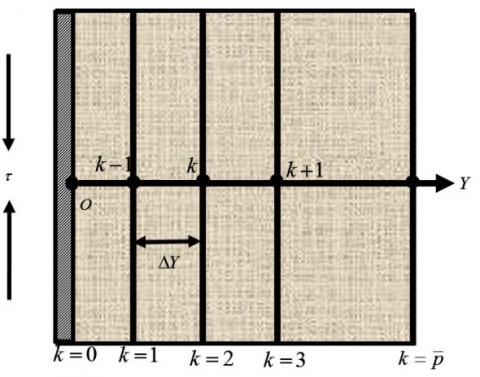Figure 2. Implicit finite difference system grid

It is assumed that the maximum length of the boundary layer is Ymax=(25) as corresponds to Y→∞ i.e. Y varies from 0 to 25 and the number of grid spacing in Y directions is $\bar{p}=(400)$, hence the uniform mesh size along Y axis becomes ΔY=0.0625 (0≤Y≤25) with a smaller time-step Δt=0.001.

Let $U^{\bar{n}}, C^{\bar{n}}$ and $T^{\bar{n}}$ denote the values of $\mathrm{U}, \mathrm{C}$ and $T$ at the end of a time-step, respectively. Using the finite difference approximation, the system of partial differential equations (7)-(9), the initial conditions (10), and the boundary conditions $(11),$ we obtain an appropriate set of the following finite difference equations;

$\frac{U_{k}^{\bar{n}+1}-U_{k}^{\bar{n}}}{\Delta \tau}-\lambda \frac{U_{k+1}^{\bar{n}}-U_{k}^{\bar{n}}}{\Delta Y}=\frac{U_{k+1}^{\bar{n}}-2 U_{k}^{\bar{n}}+U_{k-1}^{\bar{n}}}{(\Delta Y)^{2}}$

$-M U_{k}^{\bar{n}}-\gamma U_{k}^{\bar{n}}+G_{r} \bar{T}_{k}^{\bar{n}}+G_{m} \bar{C}_{k}^{\bar{n}}$

$\frac{C_{k}^{\bar{n}+1}-C_{k}^{\bar{n}}}{\Delta \tau}-\lambda \frac{C_{k+1}^{\bar{n}}-C_{k}^{\bar{n}}}{\Delta Y}=\frac{1}{S_{c}} \frac{C_{k+1}^{\bar{n}}-2 C_{k}^{\bar{n}}+C_{k-1}^{\bar{n}}}{(\Delta Y)^{2}}$

$+S_{r} \frac{T_{k+1}^{\bar{n}}-2 T_{k}^{\bar{n}}+T_{k-1}^{\bar{n}}}{(\Delta Y)^{2}}$     (12)

$\frac{C_{k}^{\bar{n}+1}-C_{k}^{\bar{n}}}{\Delta \tau}-\lambda \frac{C_{k+1}^{\bar{n}}-C_{k}^{\bar{n}}}{\Delta Y}=\frac{1}{S_{c}} \frac{C_{k+1}^{\bar{n}}-2 C_{k}^{\bar{n}}+C_{k-1}^{\bar{n}}}{(\Delta Y)^{2}}$

$+S_{r} \frac{T_{k+1}^{\bar{n}}-2 T_{k}^{\bar{n}}+T_{k-1}^{\bar{n}}}{(\Delta Y)^{2}} \frac{T_{k}^{\bar{n}+1}-T_{k}^{\bar{n}}}{\Delta \tau}-\lambda \frac{T_{k+1}^{\bar{n}}-T_{k}^{\bar{n}}}{\Delta Y}=$

$\frac{1}{P_{r}}\left[\sigma\left(\frac{T_{k+1}^{\bar{n}}-T_{k}^{\bar{n}}}{\Delta Y}\right)^{2}+(1+\sigma) \frac{\bar{T}_{k+1}^{\bar{n}}-2 \bar{T}_{k i}^{\bar{n}}+\bar{T}_{k-1}^{\bar{n}}}{(\Delta Y)^{2}}\right]$   (13)

$+D_{u} \frac{C_{k+1}^{\bar{n}}-2 C_{k}^{\bar{n}}+C_{k-1}^{\bar{n}}}{(\Delta Y)^{2}}+M E_{c}\left(U_{k}^{\bar{n}}\right)^{2}$

$+E_{c}\left(\frac{U_{k+1}^{\bar{n}}-U_{k}^{\bar{n}}}{\Delta Y}\right)^{2}$    (14)

with the resultant initial and boundary conditions are

$U_{0}^{0}=0, C_{0}^{0}=0, T_{0}^{0}=0$     (15)

$U_{0}^{\bar{n}}=1, C_{0}^{\bar{n}}=1, T_{0}^{\bar{n}}=1$

$U_{L}^{\bar{n}}=0, C_{L}^{\bar{n}}=0, T_{L}^{\bar{n}}=0_{\text {where }} L \rightarrow \infty$    (16)

Here the subscript $k$ designates the grid points with $Y$ coordinate, and the superscript $\bar{n}$ represents a value of time, $\tau=\bar{n} \Delta \tau .$ where $\bar{n}=0,1,2, \ldots .$ The velocity $(U),$ concentration (C) and temperature ( $T$ ) distributions at all interior nodal points may be computed by successive applications of the above finite difference equations. The obtained values are realistically shown in Figures 3-28.

5. Numerical Results and Discussion

To obtain the steady-state solutions, the computations have been carried out up to dimensionless time τ=50. Figure 3 represents the velocity profiles at several times. The area enclosed by a curve with both axes represents as:

$U_{A}=\int_{0}^{25} U d Y$    (17)

Similarly, for concentration and temperature profile it can be written as:

$C_{A}=\int_{0}^{25} U d Y$    (18)

$T_{A}=\int_{0}^{25} T d Y$    (19)

## 3.pngFigure 3. Velocity profiles for several values of dimensionless time, τ

## 4.pngFigure 4. Areas vs. dimensionless time, τ

Figure 4 shows the effects of the areas vs. time. In this figure it can be concluded that the results of the calculations show little changes in the quantities mentioned above after dimensionless time, τ=20 Thus the solutions for dimensionless time τ=20 are essentially steady-state solutions. To observe the physical condition of the problem, the steady-state solutions have been illustrated in Figures 5-28. The influence of Grashof number Gr on the velocity and temperature distributions are presented in Figures 5-6. It is observed that velocity and temperature profiles are increasing with the increase of Gr. The effect of Modified Grashof number Gm on the velocity and temperature distributions are described in Figures 7-8. It is observed that velocity and temperature profiles are increasing with the rise of Gm. The consequence of Prandtl number Pr on the velocity and temperature distributions are presented in Figures 9-10. It is shown that velocity and temperature profiles are decreasing with the increase of Pr. The impact of Dufour number Du on the velocity and temperature distributions are discussed in Figures 11-12. It is observed that velocity and temperature profiles show increasing effect with the increase of Du. The effect of Schmidt number Sc on the velocity and concentration distributions are presented in Figures 13-14. It is observed that velocity and concentration profiles are decreased with the increase of Sc.

The influence of Soret number Sr on the velocity, temperature distributions, and concentration distributions are discussed in Figures 15-17. It is observed that velocity, temperature, and concentration profiles show an increasing effect with the increase of Sr. The effect of Magnetic parameter M on the velocity and temperature distributions are presented in Figures 18-19. It is observed that velocity and temperature profiles show a decreasing effect with the rise of M. It is clearly seen from Figure 18 that the effects of increasing the magnetic field strength on the momentum boundary layer thickness lead to a decrease in the velocity width is because the magnetic field results in a damping effect on the velocity by creating a drug force that opposes the field motion.

## 5.pngFigure 5. Velocity profiles for several values of Grashof number, Gr

## 6.png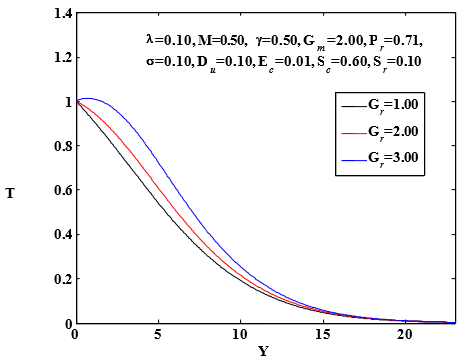Figure 6. Temperature profiles for several values of Grashof number, Gr

## 7.pngFigure 7. Velocity profiles for several values of Modified Grashof number, Gm

## 8.png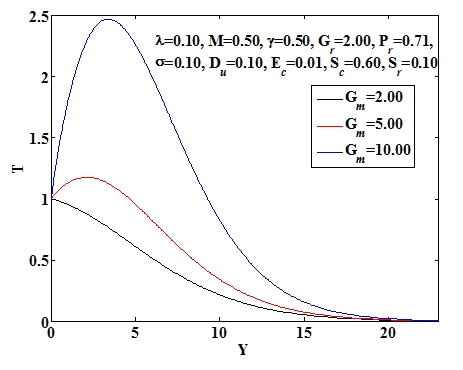Figure 8. Temperature profiles for several values of Modified Grashof number, Gm

## 9.png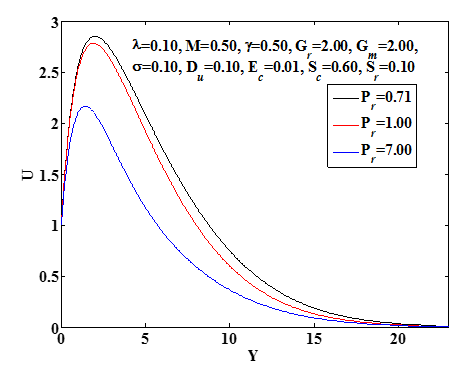Figure 9. Velocity profiles for several values of Prandtl number, Pr

## 10.pngFigure 10. Temperature profiles for several values of Prandtl number, Pr

## 11.pngFigure 11. Velocity profiles for several values of Dufour number, Du

## 12.pngFigure 12. Temperature profiles for several values of Dufour number, Du

## 13.png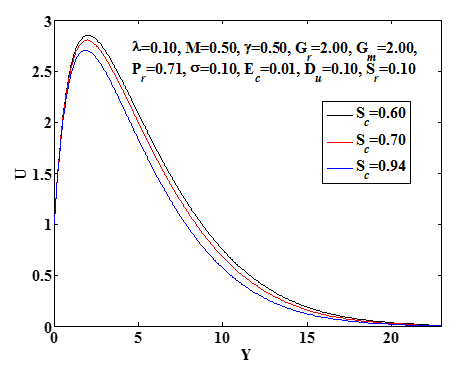Figure 13. Velocity profiles for several values of Schmidt number, Sc

## 14.pngFigure 14: Concentration profiles for various values of Schmidt number, Sc

## 15.pngFigure 15. Velocity profiles for several values of Soret Number, Sr

## 16.png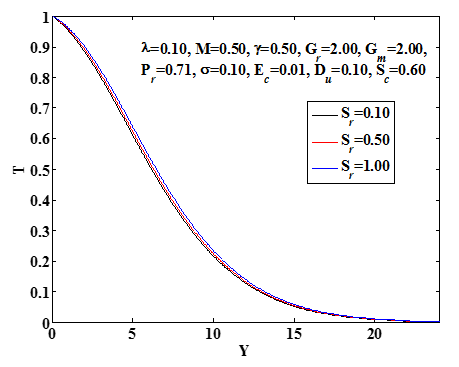Figure 16. Temperature profiles for several values of Soret Number, Sr

## 17.png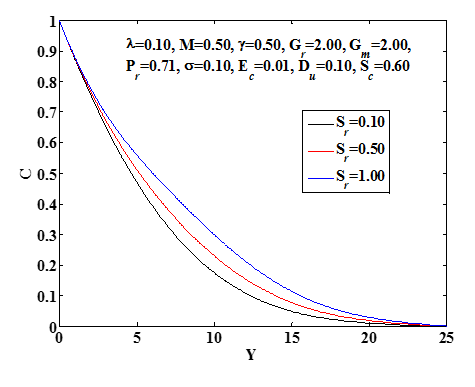Figure 17. Concentration profiles for various values of Soret Number, Sr

## 18.pngFigure 18. Velocity profiles for several values of Magnetic parameter, M

The influence of the Permeability of the porous medium γ on the velocity and temperature distributions are presented in Figures 20-21. It is shown that velocity and temperature profiles are increasing with the increase of γ. The influence of Suction parameter, γ on the velocity, temperature distributions and concentration distribution are described in Figures 22-24. It is observed that velocity, temperature, and concentration profiles are decreased with the increase of λ. The effect of Eckert number Ec on the velocity and temperature are presented in Figures 25-26. It is observed that velocity and temperature profiles are increased with the increase of Ec. The influence of adjustable thermal conductivity, σ on the velocity and temperature distributions are described in Figures 27-28. It is observed that velocity and temperature profiles are increased with the increase of σ.

## 19.png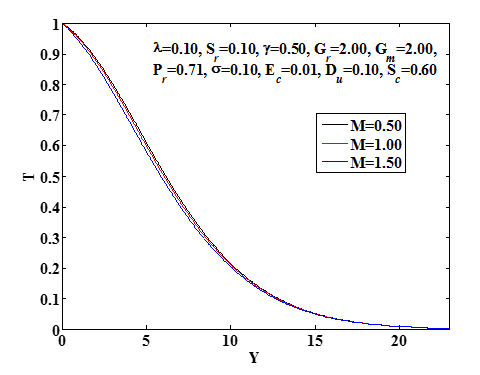Figure 19. Temperature profiles for several values of Magneticparameter, M

## 20.pngFigure 20. Velocity profiles for several values of Permeability of the porous medium, γ

## 21.pngFigure 21. Temperature profiles for several values of Permeability of the porous medium, γ

## 22.png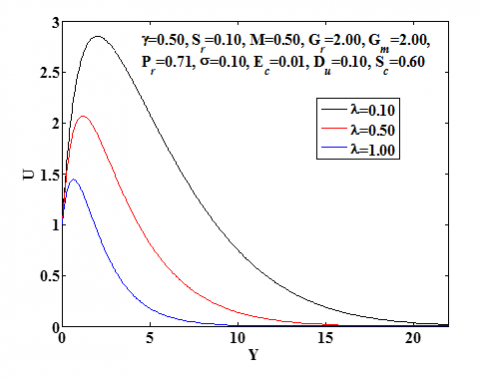Figure 22. Velocity profiles for several values of Suction Parameter, λ

## 23.pngFigure 23. Temperature profiles for several values ofSuction Parameter, λ

## 24.png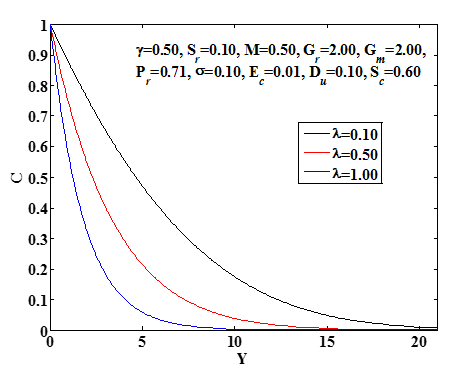Figure 24. Concentration profiles for several values ofSuction Parameter, λ

## 25.pngFigure 25. Velocity profiles for several values of Eckert number, Ec

## 26.png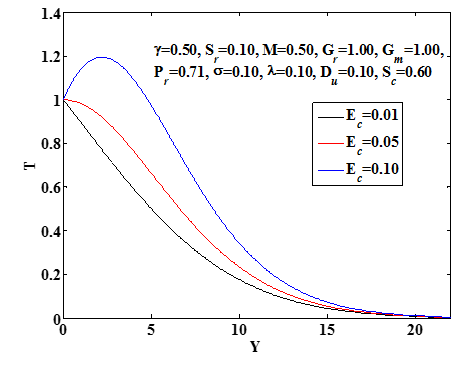Figure 26. Temperature profiles for several values of Eckert number, Ec

## 27.pngFigure 27. Velocity profiles for several values of Electrical conductivity, σ

## 28.pngFigure 28. Temperature profiles for several values of Electrical conductivity, σ

6. Conclusions

The finite-difference solution of MHD mixed convection flow through an impulsively stretched porous vertical plate with diffusion-thermo and thermal-diffusion effects have been investigated.

Some significant findings of this investigation are listed below;

(1)           The fluid velocity increases with the increase of Grashof Number, Modified Grashof Number, Dufour Number, Soret Number, Eckert Number, and Variable thermal conductivity Parameter.

(2)            Reverse effects are observed with the increase of Prandtl Number, Schmidt Number, Magnetic Parameter, Permeability of the porous medium, and Suction Parameter.

(3)           The fluid temperature increases with the increase of Grashof Number, Modified Grashof Number, Dufour Number, Soret Number, Eckert Number, and Variable thermal conductivity parameter.

(4)           Opposite effects with the increase of Prandtl Number, Magnetic Parameter, Permeability of the porous medium and Suction Parameter.

(5)           The fluid concentration increases with the increase of Soret Number and opposites effects with the increase of Schmidt Number and Suction Parameter.

As the basis for many engineering and scientific applications, for study more complex problems involving the MHD flow, it is hoped that the findings of this investigation may be useful for research of movement oil or gas and water through the reservoir of an oil or gas field, in the movement of underground water or oil as well as in the filtration and water purification processes, the findings may be useful for study of flow of oil or gas and water through the reservoir of an oil or gas field. These results may also be helpful for plasma studies as well as in power engineering, geothermal energy extractions, geophysics, and astrophysics.

References

 Benjan, A., Dincer, I., Lorente, S., Miguel, A.F., Reis, A.H. (2004). Porous and complex flow structures in modern technologies. Springer, New York, NY.

 Ingham, D.B., Pop, I. (2005). Transport phenomena in Porous media. Elsevier, Oxford, UK.

 Vafai, K. (2005). Hand book of porous media. Second edition. Taylor and Francis: New York.

 Nield, D.A., Bejan, A. (2006). Convection in porous media. Springer, New York, NY, USA, Third Edition.

 Palani, G., Srikanth, U. (2009). MHD flow past a semi-infinite vertical plate with mass transfer. Nonlinear Analysis: Modelling and Control, 14(3): 345–356.

 Makinde, O.D. (2010). On MHD heat and mass transfer over a moving vertical plate with a convective surface boundary condition. The Canadian Journal of Chemical Engineering, 88(6): 983–990.

 Duwairi, H.M. (2005). Viscous and Joule heating effects on forced convection flow from radiate isothermal porous surfaces. International Journal of Numerical Methods for Heat and Fluid Flow, 15(5): 429–440. https://doi.org/10.1108/09615530510593620

  Alim, M.A., Alam, M.D., Mamun, A. (2007). Joule heating effect on the coupling of conduction with magneto hydrodynamic free convection flow from a vertical plate. Non-Linear Analysis Modeling and Control, 12(3): 307–316.

 Raptis, A.A. (1983). Effects of mass transfer on the hydromagnetic flow past a vertical limiting surface. Aatrophys. Space Sci., 92(1): 135-142. https://doi.org/10.1007/BF00653592

 Agrawal, H.L., Ram, P.C., Singh, S.S. (1980). Combined buoyancy effects of thermal and mass diffusion on MHD natural convection flows. The Canadian Journal of Chemical Engineering, 58(1): 131-133.

 Sattar, M.A. (1993). Free and forced convection boundary layer flow through a porous medium with large suction. International Journal of Energy Research, 17(1): 1–7. https://doi.org/10.1002/er.4440170102

 Mohammad, F., Masatiro, O., Abdus, S., Mohamud, A. (2005). Similarity solutions for MHD flow through vertical porous plate with suction. Journal of Computational and Applied Mechanics, 6(1): 15–25.

 Mansour, M.A., El-Anssary, N.F., Aly, A.M. (2008). Effect of chemical reaction and viscous dissipation on MHD natural convection flows saturated in porous media with suction or injection. International Journal of Applied Mathematics and Mechanics, 4(2): 60–70.

 Khaleque, T.S., Samad, M.A. (2010). Effects of radiation, heat generation and viscous dissipation on MHD free convection flow along a stretching sheet. Research Journal of Applied Sciences, Engineering and Technology, 2(4): 368–377.

 Sharma, R.P., Mishra, S.R. (2018). A mathematical model of magnetohydrodynamicmicropolar fluid motion via permeable mediawith Soret and Dufour effects. AMSE Journals, Series Modelling B, 87(4): 250-256.

 Ahmed, t., Alam, M.M. (2015). Ionized Fluid Flow on Impulsive Vertical Porous Platewith Inclined Magnetic Field. AMSE Journals-Series: Modelling B, 84(2): 105-124.

 Uwanta, I.J. (2012). Effects of chemical reaction and radiation on Heat and Mass Transfer past a semi-infinite vertical porous plate with constant mass flux and dissipation. European Journal of Scientific Research, 87(2): 190–200.

 Govardhan, K., Kishan, N., Balaswamy, B. (2012). Effect of viscous dissipation and radiation on MHD gas flow and heat and mass transfer over a stretching surface with a uniform free stream. Journal of Engineering Physics and Thermophysics, 85(4): 909–916. https://doi.org/10.1007/s10891-012-0729-3

 Jai, S. (2012). Viscous dissipation and chemical reaction effects on flow past a stretching surface in a porous medium. Advance Theory of Applied Mechanics, 5: 323–331.

 Ibrahim, S.M. (2013). Effects of mass transfer, radiation, Joule heating and viscous dissipation on steady MHD marangoni convection flow over a flat surface with suction and injection. International Journal of Engineering Mathematics, 2013: 9. https://doi.org/10.1155/2013/903818

 Eckert, E.R.G., Drake, R.M. (1974). Analysis of heat and mass transfer. MCGraw-Hill, New York, NY, USA.

 Kafousiasis, N.G., Williams, E.M. (1995). Thermal-diffusion and diffusion-Thermo effects on mixed free-forced convective and mass transfer boundary layer flow with temperature dependent viscosity. International Journal of Engineering Science, 33: 1369-1384. https://doi.org/10.1016/0020-7225(94)00132-4

 Uwanta, I.J., Asogwa, K.K., Ali, U.A. (2008). MHD fluid flow over a vertical plate with Dufour and Soret effects. International Journal of Computer Applications, 45(2): 8–16.

 Postelnicu, A. (2010). Heat and mass transfer by natural convection at a stagnation point in a porous medium considering Soret and Dufour effects. Heat and Mass Transfer, 46(8-9): 831–840. https://doi.org/10.1007/s00231-010-0633-3

 Usman, H., Uwanta, I.J. (2013). Effect of thermal conductivity on MHD Heat and Mass transfer: flow past an infinite vertical plate with Soret and Dufour effects. American Journal of Applied Mathematics, 1(3): 28–38.

 Sarada, K., Shankar, B. (2013). The effects of Soret and Dufour on an unsteady MHD free convection flow past a vertical porous plate in the presence of suction or injection. International Journal of Engineering and Science, 2(7): 13–25.

 Uwanta, I.J., Usman, H. (2014). On the influence of soret and dufour effects on MHD free convective heat and mass transfer flow over a vertical channel with constant suction and viscous dissipation. Hindawi Publishing Corporation, International Scholarly Research Notices, 2014: 1-11. http://dx.doi.org/10.1155/2014/639159

 Alam, M.S., Rahman, M.M. (1992). Dufour and Soreteffects on MHD free convective and mass transfer flow pasta vertical flat plate embedded in a porous medium. Journalof Naval Architecture and Marine Engineering, 2(1): 369-374.